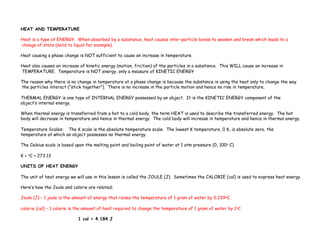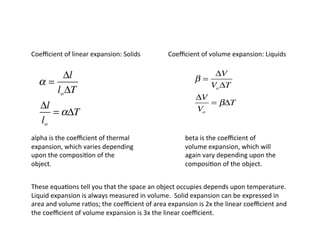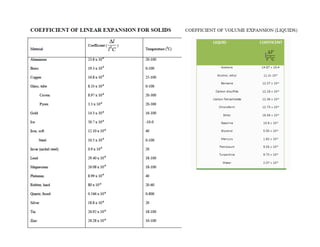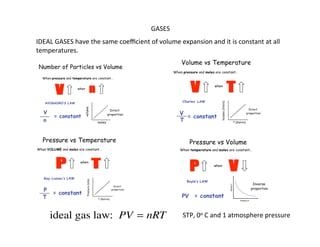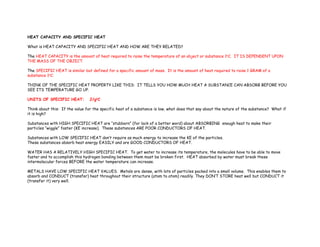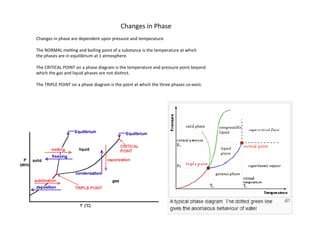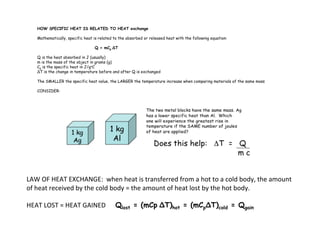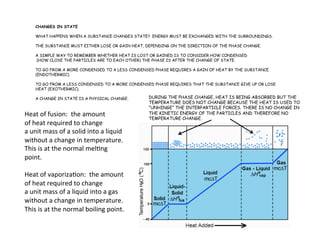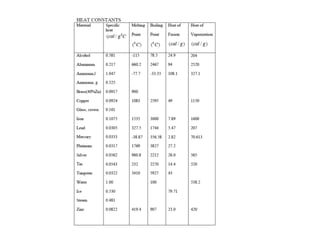1 von 902-20-08 - Thermodynamicswjerlinger4_5994635259759561834.pdfmahamedYusuf5Chapter 14 heat and heat transfer methodsSarah Sue CalbioThermodynamics - Heat and TemperatureRa JayThermoapwazap777

### Thermal energy notes Draft 1.pdf

1. HEAT AND TEMPERATURE Heat is a type of ENERGY. When absorbed by a substance, heat causes inter-particle bonds to weaken and break which leads to a change of state (solid to liquid for example). Heat causing a phase change is NOT sufficient to cause an increase in temperature. Heat also causes an increase of kinetic energy (motion, friction) of the particles in a substance. This WILL cause an increase in TEMPERATURE. Temperature is NOT energy, only a measure of KINETIC ENERGY The reason why there is no change in temperature at a phase change is because the substance is using the heat only to change the way the particles interact (“stick together”). There is no increase in the particle motion and hence no rise in temperature. THERMAL ENERGY is one type of INTERNAL ENERGY possessed by an object. It is the KINETIC ENERGY component of the object’s internal energy. When thermal energy is transferred from a hot to a cold body, the term HEAT is used to describe the transferred energy. The hot body will decrease in temperature and hence in thermal energy. The cold body will increase in temperature and hence in thermal energy. Temperature Scales: The K scale is the absolute temperature scale. The lowest K temperature, 0 K, is absolute zero, the temperature at which an object possesses no thermal energy. The Celsius scale is based upon the melting point and boiling point of water at 1 atm pressure (0, 100o C) K = oC + 273.13 UNITS OF HEAT ENERGY The unit of heat energy we will use in this lesson is called the JOULE (J). Sometimes the CALORIE (cal) is used to express heat energy. Here’s how the Joule and calorie are related: Joule (J) – 1 joule is the amount of energy that raises the temperature of 1 gram of water by 0.239oC. calorie (cal) – 1 calorie is the amount of heat required to change the temperature of 1 gram of water by 1oC. 1 cal = 4.184 J
2. Coeﬃcient  of  linear  expansion:  Solids                                  Coeﬃcient  of  volume  expansion:  Liquids   α = Δl loΔT Δl lo = αΔT alpha  is  the  coeﬃcient  of  thermal   expansion,  which  varies  depending   upon  the  composi=on  of  the     object.   β = ΔV VoΔT ΔV Vo = βΔT beta  is  the  coeﬃcient  of     volume  expansion,  which  will   again  vary  depending  upon  the   composi=on  of  the  object.   These  equa=ons  tell  you  that  the  space  an  object  occupies  depends  upon  temperature.   Liquid  expansion  is  always  measured  in  volume.    Solid  expansion  can  be  expressed  in   area  and  volume  ra=os;  the  coeﬃcient  of  area  expansion  is  2x  the  linear  coeﬃcient  and   the  coeﬃcient  of  volume  expansion  is  3x  the  linear  coeﬃcient.
3. GASES   IDEAL  GASES  have  the  same  coeﬃcient  of  volume  expansion  and  it  is  constant  at  all   temperatures.   ideal gas law: PV = nRT STP,  0o  C  and  1  atmosphere  pressure
4. HEAT CAPACITY AND SPECIFIC HEAT What is HEAT CAPACITY AND SPECIFIC HEAT AND HOW ARE THEY RELATED? The HEAT CAPACITY is the amount of heat required to raise the temperature of an object or substance 1oC. IT IS DEPENDENT UPON THE MASS OF THE OBJECT. The SPECIFIC HEAT is similar but defined for a specific amount of mass. It is the amount of heat required to raise 1 GRAM of a substance 1oC. THINK OF THE SPECIFIC HEAT PROPERTY LIKE THIS: IT TELLS YOU HOW MUCH HEAT A SUBSTANCE CAN ABSORB BEFORE YOU SEE ITS TEMPERATURE GO UP. UNITS OF SPECIFIC HEAT: J/goC Think about this: If the value for the specific heat of a substance is low, what does that say about the nature of the substance? What if it is high? Substances with HIGH SPECIFIC HEAT are “stubborn” (for lack of a better word) about ABSORBING enough heat to make their particles “wiggle” faster (KE increase). These substances ARE POOR CONDUCTORS OF HEAT. Substances with LOW SPECIFIC HEAT don’t require as much energy to increase the KE of the particles. These substances absorb heat energy EASILY and are GOOD CONDUCTORS OF HEAT. WATER HAS A RELATIVELY HIGH SPECIFIC HEAT. To get water to increase its temperature, the molecules have to be able to move faster and to accomplish this hydrogen bonding between them must be broken first. HEAT absorbed by water must break these intermolecular forces BEFORE the water temperature can increase. METALS HAVE LOW SPECIFIC HEAT VALUES. Metals are dense, with lots of particles packed into a small volume. This enables them to absorb and CONDUCT (transfer) heat throughout their structure (atom to atom) readily. They DON’T STORE heat well but CONDUCT it (transfer it) very well.
5. Changes  in  Phase   Changes  in  phase  are  dependent  upon  pressure  and  temperature   The  NORMAL  mel=ng  and  boiling  point  of  a  substance  is  the  temperature  at  which   the  phases  are  in  equilibrium  at  1  atmosphere.   The  CRITICAL  POINT  on  a  phase  diagram  is  the  temperature  and  pressure  point  beyond   which  the  gas  and  liquid  phases  are  not  dis=nct.   The  TRIPLE  POINT  on  a  phase  diagram  is  the  point  at  which  the  three  phases  co-­‐exist.
6. HOW SPECIFIC HEAT IS RELATED TO HEAT exchange Mathematically, specific heat is related to the absorbed or released heat with the following equation: Q = mCp ΔT Q is the heat absorbed in J (usually) m is the mass of the object in grams (g) Cp is the specific heat in J/goC ΔT is the change in temperature before and after Q is exchanged The SMALLER the specific heat value, the LARGER the temperature increase when comparing materials of the same mass CONSIDER: LAW  OF  HEAT  EXCHANGE:    when  heat  is  transferred  from  a  hot  to  a  cold  body,  the  amount   of  heat  received  by  the  cold  body  =  the  amount  of  heat  lost  by  the  hot  body.   HEAT  LOST  =  HEAT  GAINED            Qlost = (mCp ΔT)hot = (mCpΔT)cold = Qgain
7. CHANGES IN STATE WHAT HAPPENS WHEN A SUBSTANCE CHANGES STATE? ENERGY MUST BE EXCHANGED WITH THE SURROUNDINGS. THE SUBSTANCE MUST EITHER LOSE OR GAIN HEAT, DEPENDING ON THE DIRECTION OF THE PHASE CHANGE. A SIMPLE WAY TO REMEMBER WHETHER HEAT IS LOST OR GAINED IS TO CONSIDER HOW CONDENSED (HOW CLOSE THE PARTICLES ARE TO EACH OTHER) THE PHASE IS AFTER THE CHANGE OF STATE. TO GO FROM A MORE CONDENSED TO A LESS CONDENSED PHASE REQUIRES A GAIN OF HEAT BY THE SUBSTANCE (ENDOTHERMIC). TO GO FROM A LESS CONDENSED TO A MORE CONDENSED PHASE REQUIRES THAT THE SUBSTANCE GIVE UP OR LOSE HEAT (EXOTHERMIC). A CHANGE IN STATE IS A PHYSICAL CHANGE. Heat  of  fusion:    the  amount   of  heat  required  to  change   a  unit  mass  of  a  solid  into  a  liquid   without  a  change  in  temperature.   This  is  at  the  normal  mel=ng     point.   Heat  of  vaporiza=on:    the  amount   of  heat  required  to  change   a  unit  mass  of  a  liquid  into  a  gas   without  a  change  in  temperature.   This  is  at  the  normal  boiling  point.   DURING THE PHASE CHANGE, HEAT IS BEING ABSORBED BUT THE TEMPERATURE DOES NOT CHANGE BECAUSE THE HEAT IS USED TO “UNHINGE” THE INTERPARTICLE FORCES. THERE IS NO CHANGE IN THE KINETIC ENERGY OF THE PARTICLES AND THEREFORE NO TEMPERATURE CHANGE.